# Double Dimensional Array: Declaration, Initialization and Matrix Form

## ICSE Computer Applications

Double Dimensional Arrays builds upon the concepts of Single Dimensional Arrays. So, if you know Single Dimensional Arrays really well then understanding Double Dimensional Arrays will be very easy for you. If you don’t know about Single Dimensional Arrays then please go through its lessons first and then come back to this lesson.

The need of Double Dimensional Arrays arises from our natural instinct to arrange data in a tabular format. Most of the times when we are dealing with data, we organize it as a table. Let’s look at a few examples to make this point clear.

How does your class teacher record the marks of the entire class in all the subjects? He or she will create a table for it like this: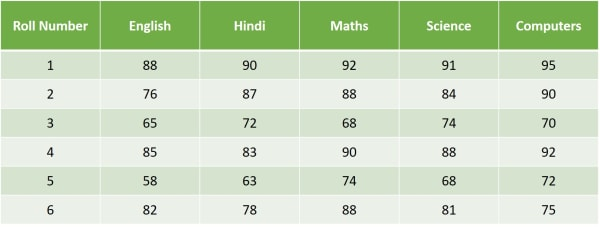How is the attendance of the class recorded in the attendance register? Again, using a table like this:If I ask you to write 3 differences between Bubble Sort and Selection sort, most probably you will list them in a tabular form like this: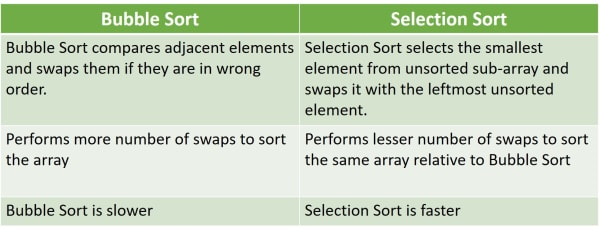I hope these examples give you a good idea about how we organize data in tables to manage it easily. Double Dimensional Arrays help us organize data as tables in our Java programs.

When I was explaining Single Dimensional Arrays, I said that you can visualize a Single Dimensional Array as a row of numbered boxes.Similarly, you can visualize a Double Dimensional Array as a group of Single Dimensional Arrays stacked upon each other like this:

In a Double Dimensional Array, we need two subscripts to refer to an element within the array. First one is for the number of rows. And second one is for the number of columns.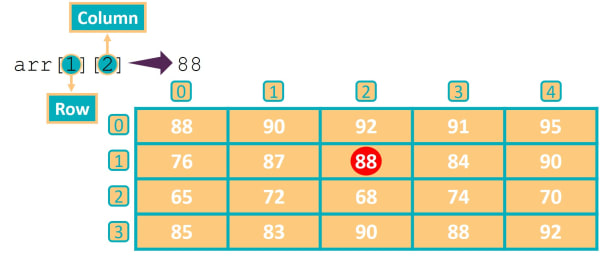As shown in the example above, to access the element with value 88, we will write arr. The first subscript is the row and the second subscript is the column.

We specify the dimensions of a Double Dimensional array as Number of rows x Number of columns. This array has 4 rows and 5 columns. So, we can write its dimensions as 4 x 5.

Like One Dimensional Arrays, all elements of a Double Dimensional array must be of the same type. Elements of different data types within a 2D array will lead to compilation errors.

## Declaring & Initializing 2D Arrays

`int arr[][] = {  {1, 3}, {5, 7}, {9, 11} };`

This statement declares `arr` as a Double Dimensional Array. `arr` contains three rows and two columns so dimensions of `arr` are 3 x 2.

Notice that there is an extra pair of square brackets in this statement. It specifies that the variable `arr` represents a Double Dimensional array.

To understand this part — `{ {1, 3}, {5, 7}, {9, 11} }` remember that a Double Dimensional Array is essentially a set of Single Dimensional Arrays, where each Single Dimensional Array forms one row of the Double Dimensional Array.

 {1, 3} 1st row {5, 7} 2nd row {9, 11} 3rd row

To initialize the Double Dimensional Array, we write the rows as Single Dimensional Arrays, separate them by commas and enclose them within a pair of curly brackets.

Like Single Dimensional Arrays, here too we can place these two pairs of square brackets between the data type and array name.

```int arr[][] = {  {1, 3}, {5, 7}, {9, 11} };
int [][] arr = {  {1, 3}, {5, 7}, {9, 11} };```

Both these statements are equivalent, you can use whichever way you like.

Let’s see a few examples of declaring and initializing 2D arrays of different data types.

```/*
a1 is an int 2D array with dimensions 3 x 2.
It can hold 3 x 2 = 6 elements.
*/
int a1[][] = {{1, 3},
{5, 7},
{9, 11}};

/*
a2 is a char 2D array with dimensions 2 x 5.
It can hold 2 x 5 = 10 elements.
*/
char a2[][] = {{‘A’, ‘E’, ‘I’, ‘O’, ‘U’},
{‘q’, ‘w’, ‘r’, ‘t’, ‘y’}};

/*
a3 is a double 2D array with dimensions 3 x 3.
It can hold 3 x 3 = 9 elements.
*/
double a3[][] = {{1.414, 1.732, 2.236},
{3.141, 2.718, 1.622},
{2.528, 5.291, 1.618}};

/*
a4 is a String 2D array with dimensions 2 x 3.
It can hold 2 x 3 = 6 elements.
*/
String a4[][] = {{“kiwi”, “pear”, “plum”},
{“milk”, “curd”, “tea”}};
```

## Displaying 2D Array Elements in Matrix Form

An important concept in Double Dimensional Arrays is to display its elements in a matrix form. Displaying elements in matrix form means displaying the elements of the Double Dimensional Array organized in rows and columns.

For example, elements of this 2D array:

`int arr[][] = {  {1, 3}, {5, 7}, {9, 11} };`

in matrix form will be displayed like this:

```1 3
5 7
9 11```

Let’s also see the matrix form of other examples that we saw earlier.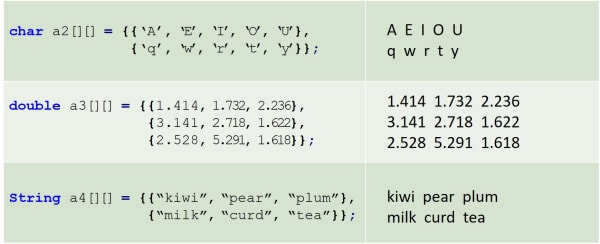### BlueJ Program to Display 2D Array in Matrix Form

```public class KboatDDA
{
public static void main(String args[]) {
int arr[][] = {{1, 3}, {5, 7}, {9, 11}};

System.out.println("2D Array Printed in Matrix Form:");
/*
* Outer loop is for the number of rows
*/
for (int i = 0; i < 3; i++) {
/*
* Inner loop is for the number of columns
*/
for (int j = 0; j < 2; j++) {
System.out.print(arr[i][j] + " ");
}
System.out.println();
}
}
}```

#### Output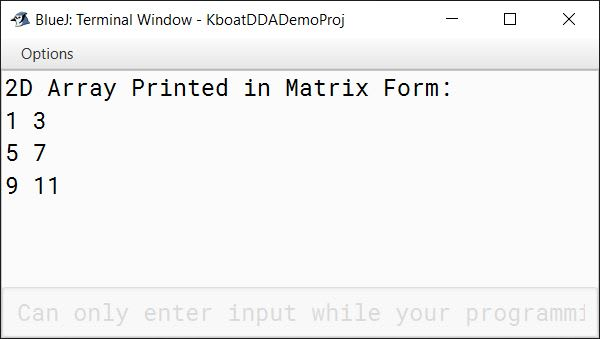In this program, we first declare and initialize `arr` as a Double Dimensional Array of type int having dimensions as 3 x 2. After that we use two nested for loops to print the elements of `arr` in matrix form. The outer loop is for the number of rows. As the rows of `arr` are numbered zero, one and two hence the outer loop runs from zero to two. Remember that in Java we start counting from 0 not 1.

The inner loop is for the number of columns. Columns of `arr` are numbered as zero and one so the inner loop runs from zero to one. Inside the inner loop we print `arr[i][j]` along with a space. Note that we have used `System.out.print` and not `System.out.println` as we want the elements of one row to be printed on the same line. Once the inner loop completes executing, one row of the array is printed.

The `System.out.println` statement after the inner loop will start the next row from a new line. Finally, as you can see in the output, the program prints the Double Dimensional Array correctly in matrix form.